Next: Trigonometrics and complex arguments Up: Modular mappings Previous: Average number of different

## Exponentials, ex

Next we will add exponentials to our repertoire of expressions for which we can compute signatures. The signature of ex will be computed as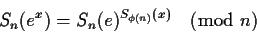where Sn(e) is arbitrarily chosen to be a primitive root (mod n) and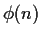is Euler's totient function.

Over rational expressions of polynomials, the only relations that S(ea) has to follow are: S(e0) = 1 and S(ea+b) = S(ea) S(eb). These are satisfied with the above definition.

S(ea) should also satisfy a negative condition, which is that there is no relation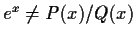for any finite-degree polynomials P(x) and Q(x). This can be proved over the reals by showing that the expansion of Q(x)ex will have a term with degree higher than the degree of P(x), and hence for sufficiently large x, |Q(x)ex| > |P(x)|.

This property should also be maintained over (mod n). To prove this we make use of the operator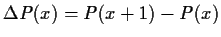. Now, each time that we apply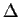to a polynomial, we reduce its degree by one. So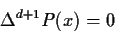where d is the degree of P(x).applied to powers (mod n) gives: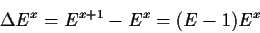or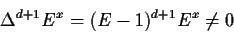Suppose that P(x) and Ex are claimed to be identical, then if the degree of P(x) is not higher than n-2, we compute the sequence P(0), P(1), ... , P(d+2). The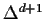of this sequence is 0, and if it were equal to the sequence E0, E1, ... Ed+1 it would be non-zero, so there cannot be a polynomial that has identical signatures to Ex. The extension to rational polynomials is by observing that the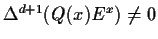too.

Choosing S(e) to be a primitive root guarantees that the range of the signatures S(ex) is as large as possible, i.e. there will bedifferent possible values for the signature. An extremely small range would force us to reconsider the probability bounds, in particular if we were computing with polynomials on exponentials.Next: Trigonometrics and complex arguments Up: Modular mappings Previous: Average number of different
Gaston Gonnet
1999-07-04